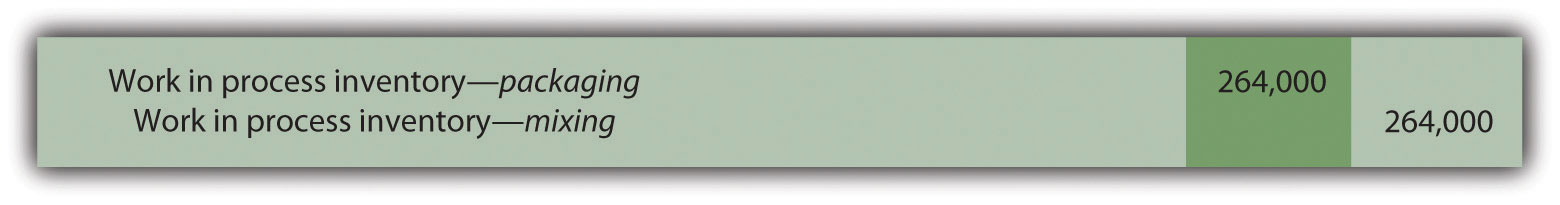## 4.4 The Weighted Average Method

### Learning Objective

1. Use four steps to assign costs to products using the weighted average method.

Most companies use either the weighted average or first-in-first-out (FIFO) method to assign costs to inventory in a process costing environment. The weighted average methodA method of process costing that includes costs in beginning inventory and current period costs to establish an average cost per unit. includes costs in beginning inventory and current period costs to establish an average cost per unit. The first-in-first-out (FIFO)A method of accounting for product costs that assumes that the first units completed within a processing department are the first units transferred out; beginning inventory costs are maintained separately from current period costs. method keeps beginning inventory costs separate from current period costs and assumes that beginning inventory units are completed and transferred out before the units started during the current period are completed and transferred out. We focus on the weighted average approach here and leave the discussion of the FIFO method to more advanced cost accounting textbooks.

Question: The primary goal stated in Chapter 2 "How Is Job Costing Used to Track Production Costs?" and Chapter 3 "How Does an Organization Use Activity-Based Costing to Allocate Overhead Costs?", and continued in this chapter, is to assign product costs to products. In a process costing system, cost per equivalent unitThe average unit cost for each product. is the term used to describe the average unit cost for each product. How is the concept of cost per equivalent unit used to assign costs to (1) completed units transferred out and (2) units still in work-in-process (WIP) inventory at the end of the period?

Answer: Costs are assigned to completed units transferred out and units in ending WIP inventory using a four-step process. We list the four steps in the following and then explain them in detail. Review these steps carefully.

Step 1. Summarize the physical flow of units and compute the equivalent units for direct materials, direct labor, and overhead.

Step 2. Summarize the costs to be accounted for (separated into direct materials, direct labor, and overhead).

Step 3. Calculate the cost per equivalent unit.

Step 4. Use the cost per equivalent unit to assign costs to (1) completed units transferred out and (2) units in ending WIP inventory.

## The Four Key Steps of Assigning Costs

Recall that Desk Products, Inc., has two departments—Assembly and Finishing. Although this chapter focuses on the Assembly department, the Finishing department would also use the four steps to determine product costs for completed units transferred out and ending WIP inventory. Table 4.2 "Production Information for Desk Products’ Assembly Department" presents information for the Assembly department at Desk Products for the month of May. Review this information carefully as it will be used to illustrate the four key steps.

Table 4.2 Production Information for Desk Products’ Assembly Department

Assembly Department—Month of May
• The company had 3,000 units in beginning WIP inventory; all were completed and transferred out during May.
• During May, 6,000 units were started. Of the 6,000 units started:

• 1,000 units were completed and transferred out to the Finishing department (100 percent complete with respect to direct materials, direct labor, and overhead); thus 1,000 units were started and completed during May.
• 5,000 units were partially completed and remained in ending WIP inventory on May 31 (60 percent complete for direct materials, 30 percent complete for direct labor, and 30 percent complete for overhead, which is applied based on direct labor hours).
• Costs in beginning WIP inventory totaled $161,000 (=$95,000 in direct materials + $40,000 in direct labor +$26,000 in overhead).
• Costs incurred during May totaled $225,000 (=$115,000 in direct materials + $70,000 in direct labor +$40,000 in overhead).

Question: Costs for the Assembly department totaled $386,000 for the month of May ($386,000 = $161,000 in beginning WIP inventory +$225,000 incurred during May). How much of the $386,000 should be assigned to (1) completed units transferred out to the Finishing department and (2) units remaining in the Assembly department ending WIP inventory? Answer: Let’s use the four key steps as follows to answer this question. Step 1. Summarize the physical flow of units and compute the equivalent units for direct materials, direct labor, and overhead. This step uses the basic cost flow equation presented in Chapter 2 "How Is Job Costing Used to Track Production Costs?" to identify the physical flow of units (the basic cost flow equation applies to costs and to units): Question: What are the two categories used to summarize the physical flow of units? Answer: The first category, units to be accounted for, includes the beginning balance (BB) and transfers in (TI). The second category, units accounted for, includes the ending balance (EB) and transfers out (TO). As you can see from the previous equation, units to be accounted for must equal units accounted for. Here is how it looks for the Assembly department for the month of May: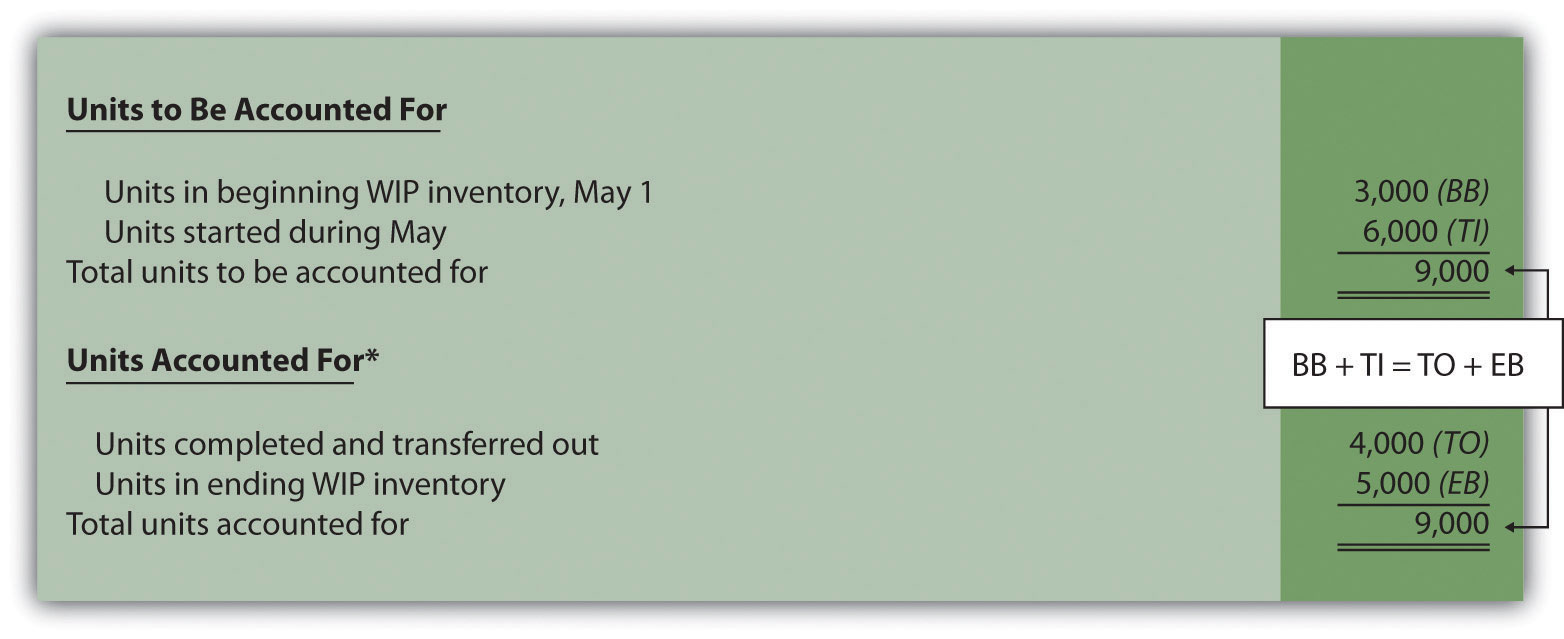*This information is used in the physical units column of Figure 4.4 "Flow of Units and Equivalent Unit Calculations for Desk Products’ Assembly Department". This step shows that 3,000 units were in WIP inventory on May 1 and 6,000 units were started during May. Thus 9,000 units must be accounted for. These 9,000 units will end up in one of two places, either completed and transferred out (to the Finishing department) or not completed and therefore in ending WIP inventory. The previous schedule shows that 4,000 units were completed and transferred out (3,000 from beginning WIP inventory and 1,000 from the units started and completed during the month), and 5,000 units remain in ending WIP inventory. Question: Based on the previous information for Desk Products, Inc., we now know that 4,000 units were completed and transferred out, and 5,000 units were in ending WIP inventory at the end of May. How do we convert this information into equivalent units? Answer: The units accounted for (4,000 transferred out and 5,000 in ending WIP inventory) must be converted into equivalent units for direct materials, direct labor, and overhead, as shown in Figure 4.4 "Flow of Units and Equivalent Unit Calculations for Desk Products’ Assembly Department". The 4,000 units transferred out are 100 percent complete for direct materials, direct labor, and overhead (otherwise, they would not be transferred out), which results in equivalent units matching the physical units. However, the 5,000 units in ending WIP inventory are at varying levels of completion for direct materials, direct labor, and overhead, and must be converted into equivalent units using the following formula (as described earlier in the chapter): Equivalent units = Number of physical units × Percentage of completion Later in step 3, we will use equivalent unit information for the Assembly department to calculate the cost per equivalent unit. Figure 4.4 Flow of Units and Equivalent Unit Calculations for Desk Products’ Assembly Department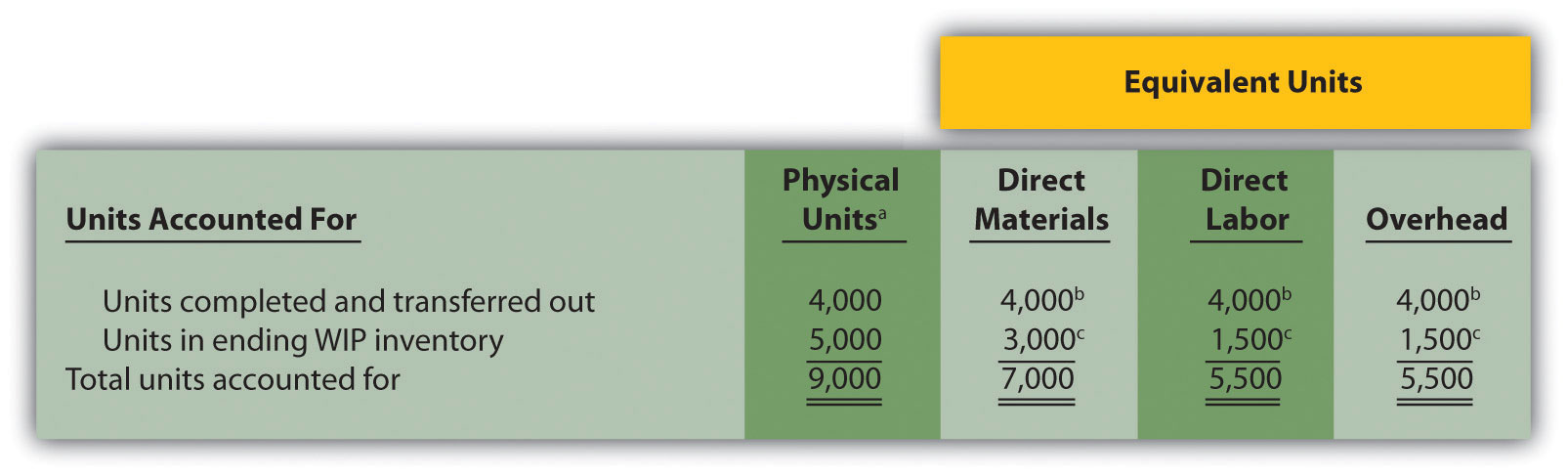a This column represents actual physical units accounted for before converting to equivalent units. b Equivalent units = Number of physical units × Percentage of completion. Units completed and transferred out are 100 percent complete. Thus equivalent units are the same as the physical units. (Information is from Table 4.2 "Production Information for Desk Products’ Assembly Department".) c Equivalent units = Number of physical units × Percentage of completion. For direct materials, 3,000 equivalent units = 5,000 physical units × 60 percent complete; for direct labor and overhead, 1,500 equivalent units = 5,000 physical units × 30 percent complete. (Information is from Table 4.2 "Production Information for Desk Products’ Assembly Department".) Step 2. Summarize the costs to be accounted for (separated into direct materials, direct labor, and overhead). Question: How do we summarize the costs that are used to calculate the cost per equivalent unit? Answer: The total costs to be accounted for include the costs in beginning WIP inventory and the costs incurred during the period. Figure 4.5 "Summary of Costs to Be Accounted for in Desk Products’ Assembly Department" shows these costs for the Assembly department. Notice that the costs are separated into direct materials, direct labor, and overhead. Figure 4.5 Summary of Costs to Be Accounted for in Desk Products’ Assembly Department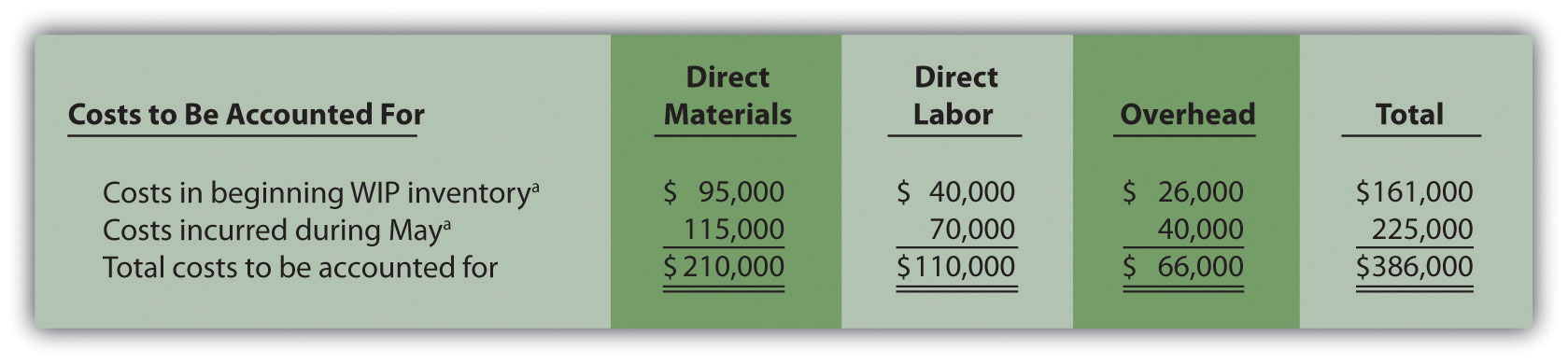a Information is from Table 4.2 "Production Information for Desk Products’ Assembly Department". Figure 4.5 "Summary of Costs to Be Accounted for in Desk Products’ Assembly Department" shows that costs totaling$386,000 must be assigned to (1) completed units transferred out and (2) units in ending WIP inventory.

Step 3. Calculate the cost per equivalent unit.

Question: We now have the costs (Figure 4.5 "Summary of Costs to Be Accounted for in Desk Products’ Assembly Department") and equivalent units (Figure 4.4 "Flow of Units and Equivalent Unit Calculations for Desk Products’ Assembly Department") needed to determine the cost per equivalent unit for direct materials, direct labor, and overhead. How do we use this information to calculate the cost per equivalent unit?

Answer: The formula to calculate the cost per equivalent unit using the weighted average method is as follows:

### Key Equation

In summary, the same formula is as follows:

Figure 4.6 "Calculation of the Cost per Equivalent Unit for Desk Products’ Assembly Department" presents the cost per equivalent unit calculation for Desk Products’ Assembly department.

Figure 4.6 Calculation of the Cost per Equivalent Unit for Desk Products’ Assembly Department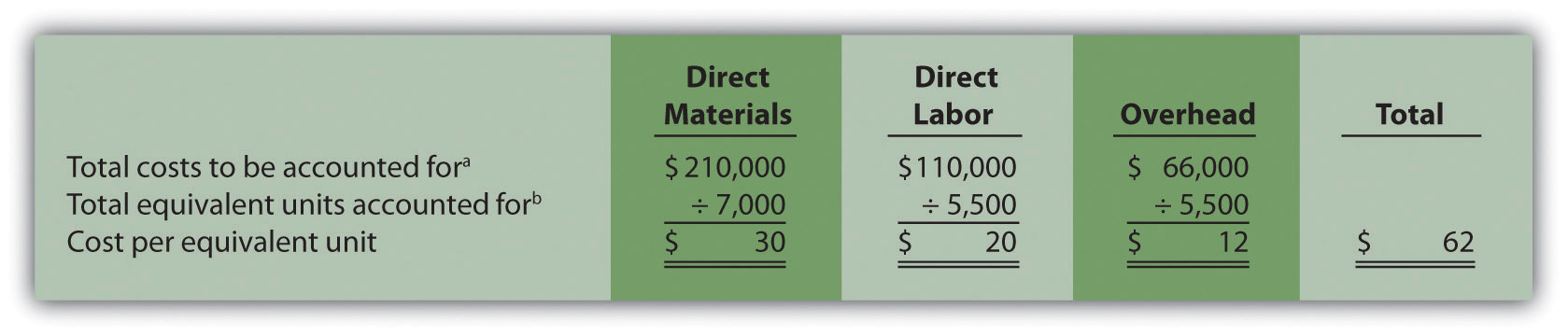The cost per equivalent unit is calculated for direct materials, direct labor, and overhead. Simply divide total costs to be accounted for by total equivalent units accounted for. It is important to note that the information shown in Figure 4.6 "Calculation of the Cost per Equivalent Unit for Desk Products’ Assembly Department" allows managers to carefully assess the unit cost information in the Assembly department for direct materials, direct labor, and overhead. We discuss this further later in the chapter.

Step 4. Use the cost per equivalent unit to assign costs to (1) completed units transferred out and (2) units in ending WIP inventory.

Question: Recall our primary goal of assigning costs to completed units transferred out and to units in ending WIP inventory. How do we accomplish this goal?

Answer: Costs are assigned by multiplying the cost per equivalent unit (shown in Figure 4.6 "Calculation of the Cost per Equivalent Unit for Desk Products’ Assembly Department") by the number of equivalent units (shown in Figure 4.4 "Flow of Units and Equivalent Unit Calculations for Desk Products’ Assembly Department") for direct materials, direct labor, and overhead. Figure 4.7 "Assigning Costs to Products in Desk Products’ Assembly Department" shows how this is done.

Figure 4.7 Assigning Costs to Products in Desk Products’ Assembly Department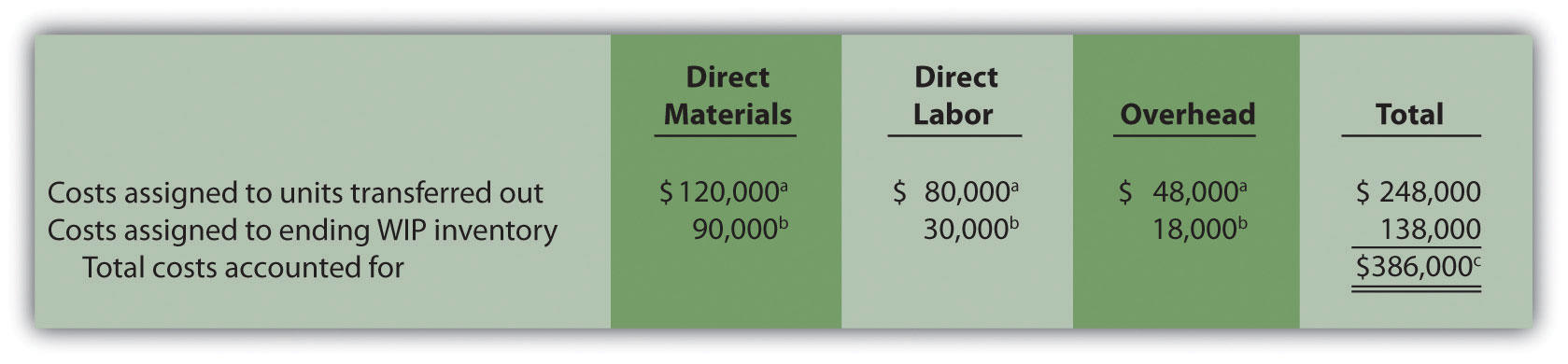a The total cost assigned to units transferred out equals the cost per equivalent unit times the number of equivalent units. For example, the cost assigned to direct materials of $120,000 = 4,000 equivalents units (Figure 4.4 "Flow of Units and Equivalent Unit Calculations for Desk Products’ Assembly Department") ×$30 per equivalent unit (Figure 4.6 "Calculation of the Cost per Equivalent Unit for Desk Products’ Assembly Department").

b The total cost assigned to units in ending inventory equals the cost per equivalent unit times the number of equivalent units. For example, the cost assigned to direct materials of $90,000 = 3,000 equivalent units (Figure 4.4 "Flow of Units and Equivalent Unit Calculations for Desk Products’ Assembly Department") ×$30 per equivalent unit (Figure 4.6 "Calculation of the Cost per Equivalent Unit for Desk Products’ Assembly Department").

c This must match total costs to be accounted for shown in Figure 4.5 "Summary of Costs to Be Accounted for in Desk Products’ Assembly Department". Although not an issue in this example, rounding the cost per equivalent unit may cause minor differences between the two amounts.

Figure 4.7 "Assigning Costs to Products in Desk Products’ Assembly Department" shows that total costs of $248,000 are assigned to units completed and transferred out and that$138,000 in costs are assigned to ending WIP inventory.

On completion of step 4, it is important to reconcile the total costs to be accounted for shown at the bottom of Figure 4.5 "Summary of Costs to Be Accounted for in Desk Products’ Assembly Department" with the total costs accounted for shown at the bottom of Figure 4.7 "Assigning Costs to Products in Desk Products’ Assembly Department". The two balances must match (note that small discrepancies may exist due to rounding the cost per equivalent unit). This reconciliation relates back to the basic cost flow equation as follows:

Although the examples in this chapter have been created in a way that minimizes rounding errors, always round the cost per equivalent unit calculations in step 3 to the nearest thousandth (e.g., if the cost per equivalent unit is $2.3739, round this to$2.374 rather than to $2). Although rounding differences still may occur, this will minimize the size of rounding errors when attempting to reconcile costs to be accounted for (step 2) with costs accounted for (step 4). ## Journalizing Costs Assigned to Units Completed and Transferred Question: Once the four-step process is complete, a journal entry must be made to record the transfer of costs out of the Assembly department and into the Finishing department. How do we record the costs associated with units completed and transferred out? Answer: At Desk Products, Inc., 4,000 units were transferred from the Assembly department to the Finishing department. Costs totaling$248,000 were assigned to these units as shown in Figure 4.7 "Assigning Costs to Products in Desk Products’ Assembly Department". The journal entry to record this at the end of May is as follows: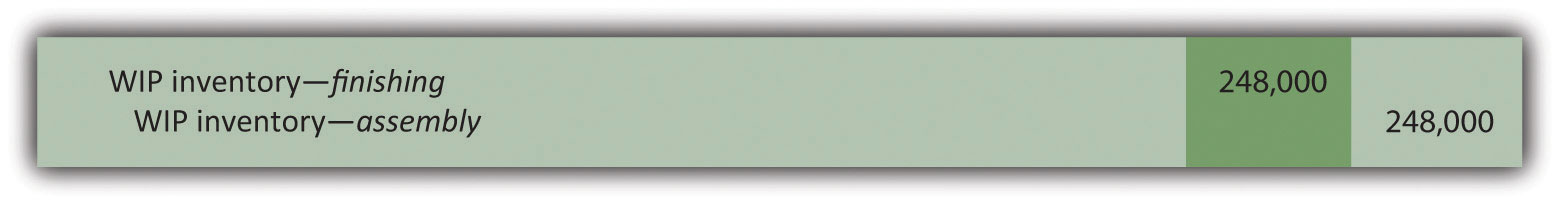(Note that this was journal entry number four, presented without dollar amounts earlier in the chapter.)

Figure 4.8 "Flow of Costs through the Work-in-Process Inventory T-Account of Desk Products’ Assembly Department" shows the flow of costs through the work-in-process inventory T-account for the Assembly department. Note that four key steps were performed for the Assembly department to determine the costs assigned to (1) completed units transferred out to the Finishing department ($248,000) and (2) units in Assembly’s WIP inventory ($138,000). Both amounts are highlighted.

Figure 4.8 Flow of Costs through the Work-in-Process Inventory T-Account of Desk Products’ Assembly Department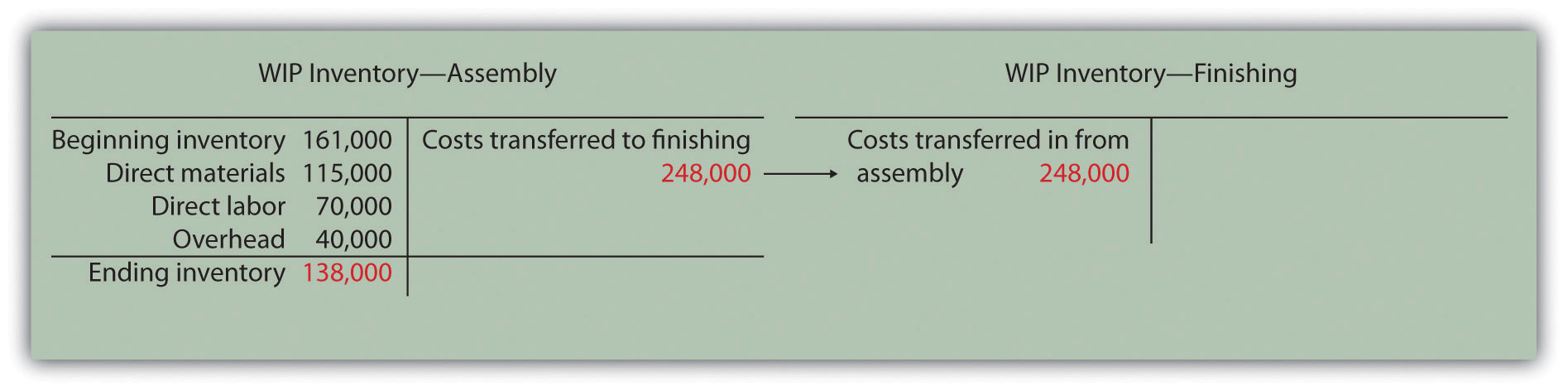The Production Process for Hershey’s Chocolate

Hershey Foods Corp. is best known for its chocolate products, including brands like Almond Joy, Hershey’s Kisses, and Reese’s. Hershey’s products are sold in more than 90 countries worldwide. According to Hershey, more than 80 million Kiss-shaped products are made every day!

Several sequential stages of production are required to produce chocolate at Hershey:

1. Fermentation. Cocoa beans are placed in large heaps for one week to allow the cocoa flavor to develop.
2. Roasting. The cocoa beans are roasted at very high temperatures.
3. Hulling. A hulling machine separates the shell from the inside of the bean (called the nib).
4. Milling. The nibs are ground into chocolate liquor (a liquid with a pure chocolate flavor that contains no alcohol).
5. Mixing. The chocolate liquor is mixed with cocoa butter, sugar, and milk. This mixture is dried into a brown powder, called chocolate crumb, and processed into chocolate paste.
6. Molding. Machines are used to fill more than 1,000 molds per minute with chocolate. The chocolate is then chilled to form solid candy.
7. Packaging. The candy is wrapped, packaged, and ready to be shipped.

Hershey likely uses a process costing system since it produces identical units of product in batches employing a consistent process. Process costing systems require the use of work-in-process inventory accounts for each process. Thus Hershey would track production costs using separate work-in-process inventory accounts for each stage of production.

### Key Takeaways

• Four steps are used to assign product costs to (1) completed units transferred out and (2) units in work-in-process inventory at the end of the period.
• The four-step process must be performed for each processing department and results in a journal entry to record the costs assigned to units transferred out.

### Review Problem 4.4

Kelley Paint Company uses the weighted average method to account for costs of production. Kelley manufactures base paint in two separate departments—Mixing and Packaging. The following information is for the Mixing department for the month of March.

• A total of 40,000 units (measured in gallons) were in beginning WIP inventory. All were completed and transferred out during March.
• A total of 70,000 units were started during March. Of the 70,000 units started,

• 20,000 units were completed and transferred out to the Packaging department (100 percent complete with respect to direct materials, direct labor, and overhead), and
• 50,000 units were partially completed and remained in ending WIP inventory on March 31 (90 percent complete for direct materials, 70 percent complete for direct labor, and 30 percent complete for overhead, which is applied based on machine hours).
• Costs in beginning WIP inventory totaled $229,000 (=$98,000 in direct materials + $41,000 in direct labor +$90,000 in overhead).
• Costs incurred during March totaled $165,000 (=$70,000 in direct materials + $35,000 in direct labor +$60,000 in overhead).

Required:

1. Use the four key steps to assign costs to units completed and transferred out and to units in ending WIP inventory for the Mixing department.
2. Prepare the journal entry necessary at the end of March to record the transfer of costs associated with units completed and transferred to the Packaging department.

Solution to Review Problem 4.4

1. The four steps are as follows:

Step 1. Summarize the physical flow of units and compute the equivalent units for direct materials, direct labor, and overhead.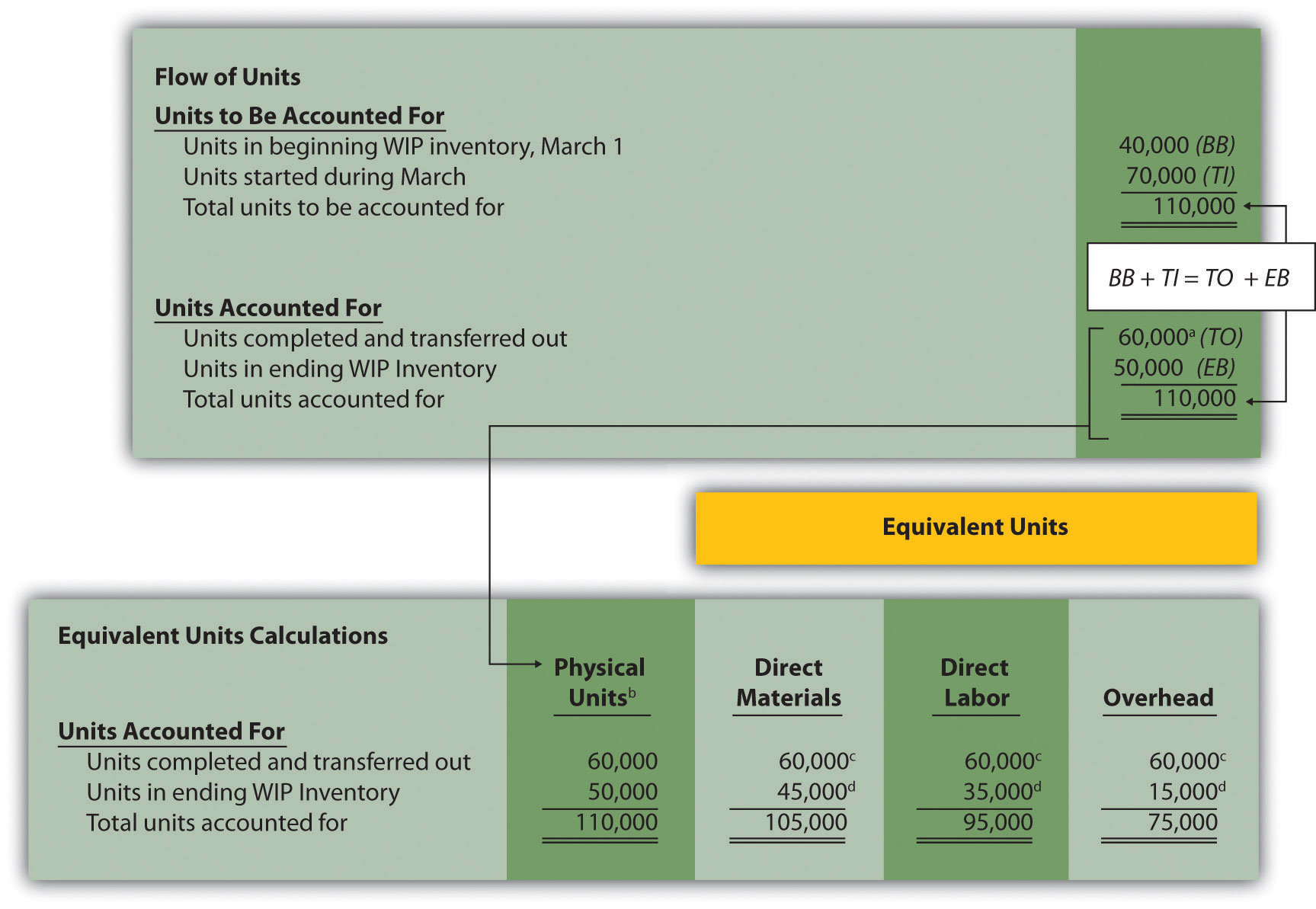a 60,000 units = 40,000 from beginning WIP inventory + 20,000 started and completed in March.

b This column represents actual physical units accounted for before converting to equivalent units.

c Equivalent units = number of physical units × percentage of completion. Units completed and transferred out are 100 percent complete. Thus equivalent units are the same as the physical units.

d Equivalent units = number of physical units × percentage of completion. For direct materials, 45,000 equivalent units = 50,000 physical units × 90 percent complete; for direct labor, 35,000 equivalent units = 50,000 physical units × 70 percent complete; for overhead, 15,000 equivalent units = 50,000 physical units × 30 percent complete.

Step 2. Summarize the costs to be accounted for (separated into direct materials, direct labor, and overhead).e Information is given.

Step 3. Calculate the cost per equivalent unit.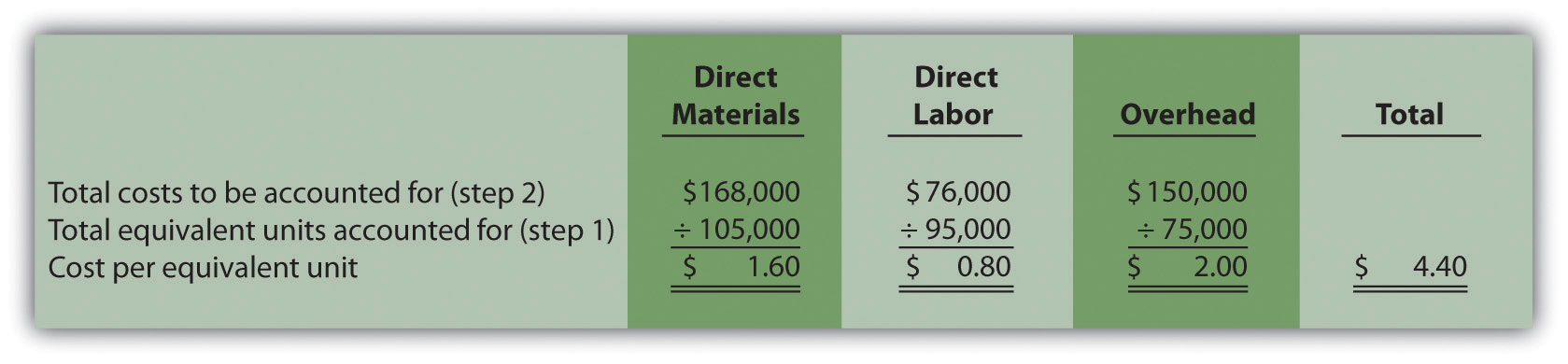Step 4. Use the cost per equivalent unit to assign costs to (1) completed units transferred out and (2) units in ending WIP inventory.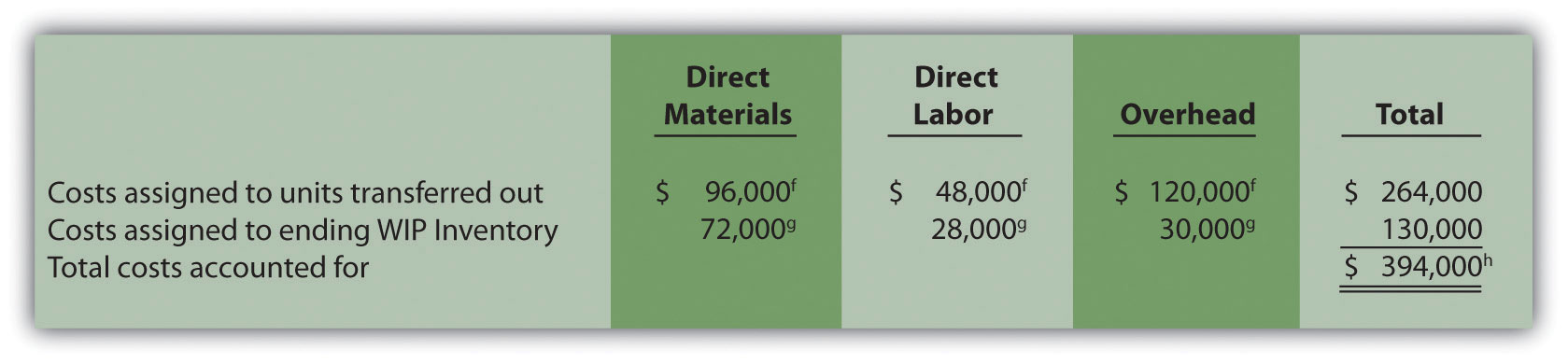f Total costs assigned to units transferred out equals the cost per equivalent unit times the number of equivalent units. For example, costs assigned for direct materials of $96,000 = 60,000 equivalents units (from step 1) ×$1.60 per equivalent unit (from step 3).

g Total costs assigned to ending WIP inventory equals the cost per equivalent unit times the number of equivalent units. For example, costs assigned for direct materials of $72,000 = 45,000 equivalent units (from step 1) ×$1.60 per equivalent unit (from step 3).

h This must match total costs to be accounted for in step 2, as shown in the following:

2. As shown in step 4, \$264,000 in total costs are assigned to units completed and transferred out. The entry to record this is as follows: